Chemistry Model Test - 2017

# Chemistry Model Test - 2017 - IIT JAM

Test Description

## 60 Questions MCQ Test IIT JAM Past Year Papers and Model Test Paper (All Branches) - Chemistry Model Test - 2017

Chemistry Model Test - 2017 for IIT JAM 2023 is part of IIT JAM Past Year Papers and Model Test Paper (All Branches) preparation. The Chemistry Model Test - 2017 questions and answers have been prepared according to the IIT JAM exam syllabus.The Chemistry Model Test - 2017 MCQs are made for IIT JAM 2023 Exam. Find important definitions, questions, notes, meanings, examples, exercises, MCQs and online tests for Chemistry Model Test - 2017 below.
Solutions of Chemistry Model Test - 2017 questions in English are available as part of our IIT JAM Past Year Papers and Model Test Paper (All Branches) for IIT JAM & Chemistry Model Test - 2017 solutions in Hindi for IIT JAM Past Year Papers and Model Test Paper (All Branches) course. Download more important topics, notes, lectures and mock test series for IIT JAM Exam by signing up for free. Attempt Chemistry Model Test - 2017 | 60 questions in 180 minutes | Mock test for IIT JAM preparation | Free important questions MCQ to study IIT JAM Past Year Papers and Model Test Paper (All Branches) for IIT JAM Exam | Download free PDF with solutions
 1 Crore+ students have signed up on EduRev. Have you?
Chemistry Model Test - 2017 - Question 1

### Silver article turns black when kept in the open for a few days due to formation of

Chemistry Model Test - 2017 - Question 2

### The decreasing order of the first ionization energy of the following elements is

Chemistry Model Test - 2017 - Question 3

### Which of these compounds are not aromatic in character ?

Chemistry Model Test - 2017 - Question 4

R- alanine is represented by______

Chemistry Model Test - 2017 - Question 5

Toluene when refluxed with bromine in the Presence of light gives mainly______

Chemistry Model Test - 2017 - Question 6

An element X on exposure to moist air turns reddish-brown and a new compound Y is formed. The substance X and Y are

Chemistry Model Test - 2017 - Question 7

If a Particle has linear momentum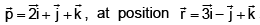then its angular momentum is ________

Detailed Solution for Chemistry Model Test - 2017 - Question 7Angular momentum
J = r × p

= (3i - j + k) × (2i + j + k)

= i(-1-1) +j(3-2) +k(3+2)

= -2i + j + 5k

Chemistry Model Test - 2017 - Question 8

The chemical formula of lead sulphate is

Chemistry Model Test - 2017 - Question 9

An electron is found in an orbital with one radial node and two angular nodes. Which orbital electron is it ?

Detailed Solution for Chemistry Model Test - 2017 - Question 9

For 4d orbital, n = 4 and l = 2
Number of radial nodes = n - l -1 = 4 - 2 - 1 = 1
Number of angular nodes =  l = 2
The number of angular and radial nodes of 4d orbital are 2 and 1 respectively.

Chemistry Model Test - 2017 - Question 10

n- simple molecule of solute A associate in solution as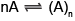The degree of association is x. what is the number of particles at equilibrium ?

Chemistry Model Test - 2017 - Question 11

The final product of the reaction is: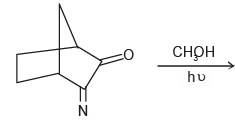Chemistry Model Test - 2017 - Question 12

In the given reaction -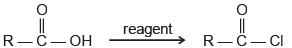reagent may be:

Chemistry Model Test - 2017 - Question 13

In the given reaction sequence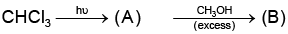(B) is _______

Detailed Solution for Chemistry Model Test - 2017 - Question 13

Chloroform when react with hv Phosgene (CCl2O).

and when phosgene react with alcohol dialkyl carbonate is produced.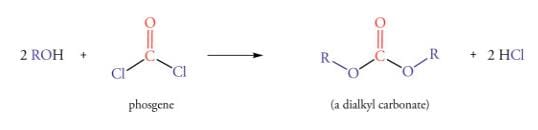Chemistry Model Test - 2017 - Question 14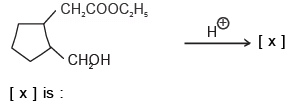Chemistry Model Test - 2017 - Question 15

In the given reaction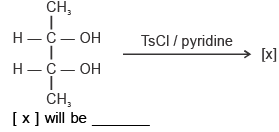Chemistry Model Test - 2017 - Question 16

In the given reaction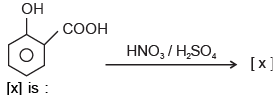Detailed Solution for Chemistry Model Test - 2017 - Question 16

Explanation : A mixture of concentrated sulphuric acid and concentrated nitric acid introduces nitro group into benzene ring in an electrophilic aromatic substitution reaction.

When phenol is treated separately with a mixture of concentrated sulphuric acid and concentrated nitric acid, 2,4,6-trinitrophenol ( also known as picric acid) is acid is obtained. Hydroxyl group is activating and ortho, para directing.

When nitrobenzene is treated separately with a mixture of concentrated sulphuric acid and concentrated nitric acid, m-dinitrobenzene is acid is obtained. Nitro group is deactivating and meta directing.

Chemistry Model Test - 2017 - Question 17

When sulphanilic acid is treated with excess of bromine water, it gives _____

Chemistry Model Test - 2017 - Question 18

Which of the following will be more reactive for E1 reaction?

Chemistry Model Test - 2017 - Question 19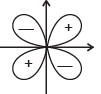The shape of which one of the orbitals corresponds to the angular wave function in the figure given above ?

Chemistry Model Test - 2017 - Question 20

The ground stable energy of hydrogen atom is - 13.6 ev what is the ground state energy of atom?

Detailed Solution for Chemistry Model Test - 2017 - Question 20

For n=1 me -13.6ev and n=2 me -3.4ev then excitation energy = final - initial energy then 10.2ev.

Chemistry Model Test - 2017 - Question 21

Taking into account the isoelectronic Principle and VSEPR Theory, predict in which one of the following pairs, both molecular species have Pyramidal geometry ?

Chemistry Model Test - 2017 - Question 22

XeF3+ belongs to which one of the following AXnEm nomenclature (A = Central, X = singly bonded liquid and E = an unshared electron pair) ?

Chemistry Model Test - 2017 - Question 23

Consider the 0.1N aqueous solutions of the following electrolytes:

1. NaOH
2. NH4OH
3. CH3COOH
4. HCl

The correct sequence of their increasing ph is

Chemistry Model Test - 2017 - Question 24

The molecule N (Sime3)3 has a

Chemistry Model Test - 2017 - Question 25

Haemoglobin a complex containing iron is a constituent of blood. The oxidation state of iron of the complex is ________

Chemistry Model Test - 2017 - Question 26

Match list- I (The metallozymes) with list- II (The metal present in the enzyme) and select the correct answer using the codes given below the lists: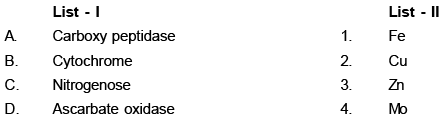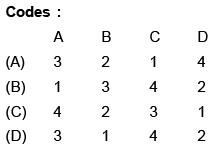Chemistry Model Test - 2017 - Question 27

The isomerism that are possible in the w(III) complexes [Co(NH3)3 (NO2/3)] and [Co(NH3)3 NO2]Cl2 respectively, are _______

Chemistry Model Test - 2017 - Question 28

For the reaction :

2Cl(g) → Cl (g)

the thermodynamic properties

Chemistry Model Test - 2017 - Question 29

For an enzyme catalysed reaction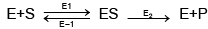Where the symbol have their usual meaning, using the steady state approximation for [ES]. The overall rate equation workout to be

Chemistry Model Test - 2017 - Question 30

The standard oxidation potential of copper and silver electrodes are -

and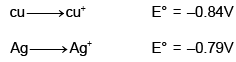Respectively, an electrochemical cell containing a copper electrode dipping in a 0.1m CuSO4 solution and a silver electrode dipping in a 0.1m AgNO3 solution is formed using a salt bridge when current is drawn from the cell.

*Multiple options can be correct
Chemistry Model Test - 2017 - Question 31

Which of the following are aromatic ?

*Multiple options can be correct
Chemistry Model Test - 2017 - Question 32

Which of the following compounds has (L) configuration ?

*Multiple options can be correct
Chemistry Model Test - 2017 - Question 33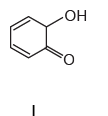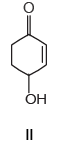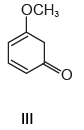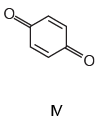Which among these can exist tautomerism ?

*Multiple options can be correct
Chemistry Model Test - 2017 - Question 34

Lysione is not used as :

*Multiple options can be correct
Chemistry Model Test - 2017 - Question 35

Which of the following is correct about ΔG ?

*Multiple options can be correct
Chemistry Model Test - 2017 - Question 36

The angular momentum of electron can have the value (s) ___

*Multiple options can be correct
Chemistry Model Test - 2017 - Question 37

The correct starting material and product of different disintegration series are______

*Multiple options can be correct
Chemistry Model Test - 2017 - Question 38

In which of the following set, both the species are isoelectronic and and isostructural?

*Multiple options can be correct
Chemistry Model Test - 2017 - Question 39

Which of the following statement is/are correct

*Multiple options can be correct
Chemistry Model Test - 2017 - Question 40

In which of these compounds does the carbon atom (underlined) nor use Sp3 hybridization for bond formation.

*Answer can only contain numeric values
Chemistry Model Test - 2017 - Question 41

How many d- electrons in Cu+ (At. No. = 29) can have the spin quantum number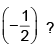*Answer can only contain numeric values
Chemistry Model Test - 2017 - Question 42

One gram of 226Rq has an activity of nearly 1 Ci. The half life of 226Ra is ____ years.

*Answer can only contain numeric values
Chemistry Model Test - 2017 - Question 43

The non- compressive volume of a gas is ____ times than the actual volume of gas molecules.

*Answer can only contain numeric values
Chemistry Model Test - 2017 - Question 44

Calculate the enthalpy of the following reaction :

H2C = CH2(g) + H2(g) → CH3 – CH3(g)

The bond energies of C- H, C- C, C=C and H- H are 99, 83, 147 and 104 kcal respectively.

*Answer can only contain numeric values
Chemistry Model Test - 2017 - Question 45

For a reaction A+B → C+D. If the concentration of A is doubled without altering the concentration of B, the rate gets doubled, if the concentration of B is increased by nine times without altering the concentration of A, the rate gets tripled. The order of reaction is _____

*Answer can only contain numeric values
Chemistry Model Test - 2017 - Question 46

For the hopothetical reactions, the equilibrium constant (k) values are given below: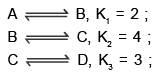The equilibrium constant (k) for the reaction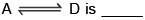*Answer can only contain numeric values
Chemistry Model Test - 2017 - Question 47

What current strength in ampere will be required to liberate 10g of chlorine from sodium chloride solution in one hour?

*Answer can only contain numeric values
Chemistry Model Test - 2017 - Question 48

How many stereoisomers exist with the following basic connectivity?

Br CH2 CH (CH3). CH2 CH3

*Answer can only contain numeric values
Chemistry Model Test - 2017 - Question 49

Use the following data to answer the question below: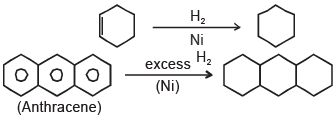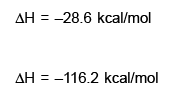The resonance energy of anthracene is _____

Detailed Solution for Chemistry Model Test - 2017 - Question 49

From first reaction we see that for hydrogenation of one double bond we need -28.6 kcal energy.And we know that anthracene contain 7 double bond .So calculated value of hydrogenation of anthracene is (7*-28.6=-200.2).

Resonance energy =Experimental -Calculated

=-116.2-(-200.2)

=84.

*Answer can only contain numeric values
Chemistry Model Test - 2017 - Question 50

Density of lithium atom is 0.53 g/cm3. The edge length of Li is 3.5 A°. Find out the number of lithium atoms is a unit cell. (N0 = 6.022 x1023/mol& M = 6.94)

*Answer can only contain numeric values
Chemistry Model Test - 2017 - Question 51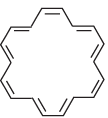What value of n in Huckel's rule applies to the aromatic compound shown above?

*Answer can only contain numeric values
Chemistry Model Test - 2017 - Question 52

When methyl glucopyranoside reacts with periodic acid, how many moles of the oxidising agent are consumed per mole of sugar ?

*Answer can only contain numeric values
Chemistry Model Test - 2017 - Question 53

How many isomeric aldehydes are possible with formula C5H10O ?

*Answer can only contain numeric values
Chemistry Model Test - 2017 - Question 54

The ionic radius of the fluoride ion is ____ using only the following interionic distance and the radius of the iodide ion 2.19A°.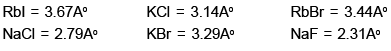*Answer can only contain numeric values
Chemistry Model Test - 2017 - Question 55

How many nearest, neighbours are there in an atom or ions for an octahedral hole of a closed packed structure ?

*Answer can only contain numeric values
Chemistry Model Test - 2017 - Question 56

In hydrogen atom, energy of first excited state is - 3.4ev, then the K.E. of same orbital of hydrogen atom is ____ev

*Answer can only contain numeric values
Chemistry Model Test - 2017 - Question 57

The molecular MLx is planar with 7 pairs of electrons around M in the valence shell, the value of x is ______.

*Answer can only contain numeric values
Chemistry Model Test - 2017 - Question 58

In the electronic structure of H2SO4, the total number of unshared electrons is ____.

*Answer can only contain numeric values
Chemistry Model Test - 2017 - Question 59

The dissociation constant of a substituted benzoic acid at 25°C is 1.0 × 10–4. The pH of a 0.01 M solution of its sodium salt is _______.

*Answer can only contain numeric values
Chemistry Model Test - 2017 - Question 60

An excess of AgNO3 is added to 100 mL of a 0.01 M solution of dichlorotetraaquachromium (III) chloride. The number of moles of AgCl precipitate would be

## IIT JAM Past Year Papers and Model Test Paper (All Branches)

8 docs|48 tests
Information about Chemistry Model Test - 2017 Page
In this test you can find the Exam questions for Chemistry Model Test - 2017 solved & explained in the simplest way possible. Besides giving Questions and answers for Chemistry Model Test - 2017, EduRev gives you an ample number of Online tests for practice

## IIT JAM Past Year Papers and Model Test Paper (All Branches)

8 docs|48 tests(Scan QR code)